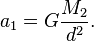# Gravity

In Universe Sandbox, two objects in a simulation will exert an attracting force on each other due to gravity. This force will affect their motion over time.

## Physics

The strength of the gravitational force depends on the mass of each object, M1 and M2, the distance between the objects, d, and the gravitational constant of the simulation, G, according to the following equation:$F = G\frac{M_1 M_2}{d^2}.$

This force will affect each object's velocity. The acceleration, or change in velocity over time, of each object depends only on the other object's mass, not its own. For example, the acceleration of object 1 due to gravity would be$a_1 = G\frac{M_2}{d^2}.$

The effects of gravity on the motion of objects in a simulation is simulated by Universe Sandbox's N-body simulation, which calculates the forces acting on each object at every time step, the accelerations that these forces would cause, and the movement of the objects as a result.

## Special Cases

For performance reasons, not all objects in Universe Sandbox simulate the effects of gravity. Objects that are non-attracting, such as fragments, will not exert a gravitational force on other objects. Object that are non-attracted, such as supernova clouds, will not feel the gravitational force of other objects.